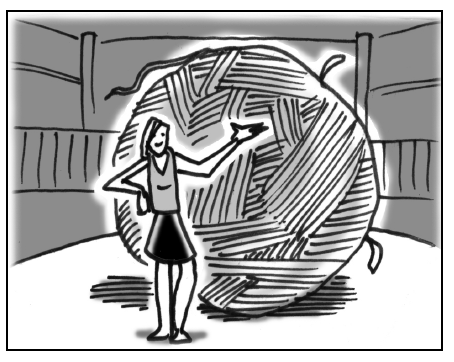### Home > GC > Chapter 12 > Lesson 12.1.1 > Problem12-6

12-6.Cawker City, Kansas, claims to have the world’s largest ball of twine. Started in 1953 by Frank Stoeber, this ball has been created by wrapping more than $1300$ miles of twine. In fact, this giant ball has a circumference of $40$ feet. Assuming the ball of twine is a sphere, find the surface area and volume of the ball of twine.

Find the radius of the ball by using the circumference.

Use the radius to find the surface area.

Use the radius to find the volume.

$\text{Volume}\approx1080.0\text{ ft}^3$